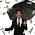Monday, February 29, 2016

Quick Tricks: Casio Programming Calculators and webcal.freetzi.com

Quick Tricks:  Casio Programming Calculators and webcal.freetzi.com

I spent the last week learning how the Casio fx-3650p can be programmed to execute amazing calculations.  The reason why I say amazing is that on the surface, the fx-3650p is limited, both in programming space (360 bytes allocated on 4 program slots) and the number of variables (7: A, B, C, D, X, Y, M).  Please check out the WebCal Page Counting Machine, run by David Chuek:

The website is in Chinese (I believe) and I am grateful for Google Translate because I am not proficient in Chinese.

* Gamma Function, Elliptic Functions (for x<1), Beta, Zeta, and other special functions
* Determinant of a 3 x 3 Matrix
* Squaring a square matrix
* 4 and 8 point Fast Fourier Transform
* Geometry:  Distance from a point to a line, area from a set of vertices
* Prime Factorization
* Engineering Programs: Snell’s Laws, Prism, Ohm’s law
* Days between Dates, Day of the Week

If you have a Casio programming calculator, check this awesome website out.

While I was learning to code the programs myself, I notice there were several tricks that were used to save space.  I want to test the tricks for the Casio fx-5800p and Casio fx-9860GII (Linear Input Mode).   The case for the fx-9860GII should be the same for other modern Casio graphing calculators (9750g, Prizm).

Quickly Setting X = 1 and Y = 0

Syntax:  Pol(1,0)
Calculators:   fx-3650p only

Note:  Pol(1,0) sets I to 1 and J to 0 for the fx-5800p.

The Power of Implied Multiplication

Most Casio programming calculators have only single-letter variables, which allows for implied multiplication.  For example, typing AB multiples the values stored in A and B.  In the examples listed below, I use variables A and B, but it applies to all the other variables.

Implied Multiplication and Square Root

Syntax:  √AB
What it calculates:  √(A*B)
Calculators: fx-3650p, fx-5800p, fx-9860GII

Example:  A = 5, B = 10;  √AB returns 7.071067812  (√50)

Implied Multiplication and Powers

Syntax:  n^AB
What it Calculates:  (n^A)*B
Calculators: fx-3650p, fx-9860GII (linear input only)

Example:  2^AB where A = 5, B = 10;  2^AB returns 320   (2^5*10)

The Jump Function Shortcuts

If the Expression is Nonzero

Syntax:  (var/expression) ⇒ (execute this statement of var/expression ≠  0) : (next command)
The next command must exist or an error occurs

Calculators:  fx-3650p, fx-5800p, fx-9860GII

Example: Ask for A and B.  Divide A by B, unless B is 0.
Prog2
? → A: ? → B: B ⇒ A ÷ B: Ans

A = 13.5, B = 2.7, result is 5
A = 13.5, B = 0, result is whatever is last stored in Ans

Using the Jump Function to create an AND condition

Connecting two or more jump functions (⇒) to create an AND condition.

Syntax – 2 Conditions:
(condition 1) ⇒ (condition 2) ⇒ (do if conditions 1 and 2 are true) : (next command)

Syntax – 3 Conditions:
(cond 1) ⇒ (cond 2) ⇒ (cond 3) ⇒ (do if all three conditions are 3) : (next command)

Example: Calculate the eccentricity of an ellipse, assuming A ≥ B.  If anything improper is entered, -1 is returned.

Prog3
? → A: ? → B: -1 → C: A ≥ B ⇒ A > 0 ⇒ B > 0 ⇒ √(1 – B2/A2 → C: C

A = 11.5, B = 3.6, result 0.949738796
A = 6, B = 8, result -1
A = -2, B = 1, result -1

Independent Memory

M+:  Calculate the expression, add to M and store the result to M
M-:  Calculate the expression, subtract from M and store the result to M

Syntax:  (expression) M+,  (expression) M-
Calculators: fx-3650p, fx-5800p.
Note:  This should work on any Casio calculator with M+ and M- available on the keyboard.

Example:
Store 7 in M.
3^2 + 4 M+ returns 13 in the display, with M = 20
2^3 – 1 M- returns in the display, with M = 13

Absolute Value

Does you calculator not have an absolute value function?  Try this trick:

Syntax: √A2
What it calculates:  |A|
Calculators:  fx-3650p, fx-5800p, fx-9860GII

Examples:  A = 55, B = -55
√A2 returns 55
√B2 returns 55

Extracting the Integer Part of a Number when the Function Int/Intg is not available

Two ways to do it:
* Syntax:  Fix 0:  Rnd:  Norm 1
* Syntax:  Switch the fx-3650p to Base Mode, 3 for Decimal, then enter your program

The Last Answer (Ans) skips over a prompt

Syntax:  (calculation):  ? → var:  f(Ans)
Calculators: fx-3650p, fx-5800p, fx-9860GII

Prog1
5^1.5 : ? → A:  Ans + A
Let A = 2, the result is 13.18033989
Let A = 9.75, the result is 20.93033989

Happy Leap Year Day!   Talk to you next time,

Eddie

This blog is property of Edward Shore.  2016

1.This comment has been removed by the author.

2.U r right,I tried with Power of Implied Multiplication in casio programming calculator, it has only one variable,its single variable if you enter 2 variable,it will take as one variable,read more @ http://www.moderncalculator.com

3.So how do you save space on the casio fx 3650 and save more functions by using this tricks?

HP 42S and TI-60: Dimensions of a Race Track

HP 42S and TI-60:  Dimensions of a Race Track We have a race track that consists of two rectangular tracks connected by a circular ri...